## Day 57: Capacitor-Controlled Tone Generator

The frequency generated by the capacitance measuring circuit is between 1 and 10 kHz for the capacitances that we are measuring. At the AP Summer Institute, Patrick Polley suggested driving a speaker with this circuit. I had some time after school yesterday, so I added an NPN transistor to the circuit since the 555 doesn’t provide enough current to drive a speaker. Today at the start of class, I shared the modified circuit with my students. As students pressed on the parallel plate capacitor, the generated tone changed; it became a simple musical instrument!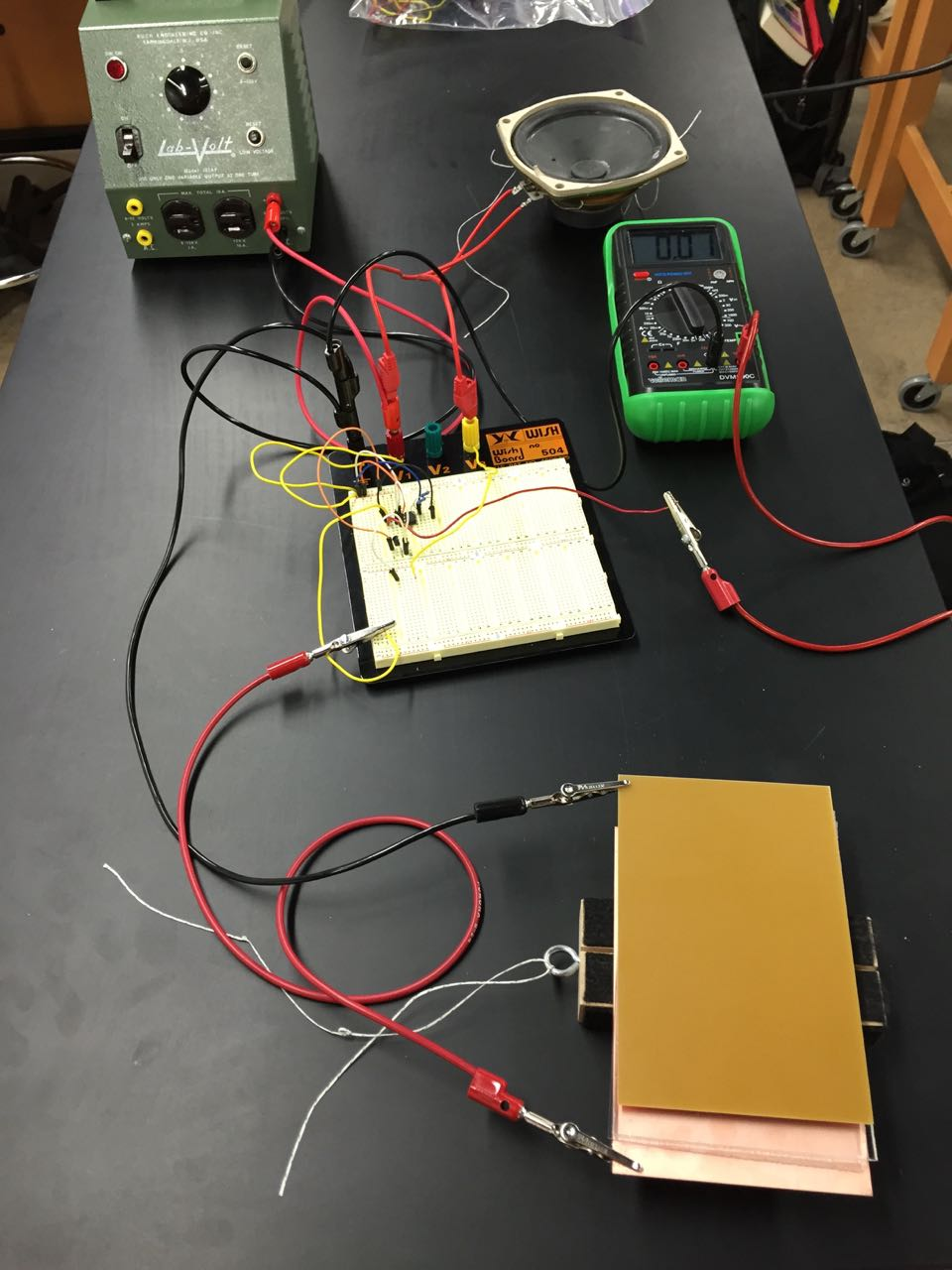##electrostatics

## Day 56: Capacitance

Today in AP Physics 2, we discussed the results of the capacitor paradigm lab. The graphs clearly showed an inverse relationship between capacitance and distance. The plot of capacitance vs. area was linear, but not as good a fit.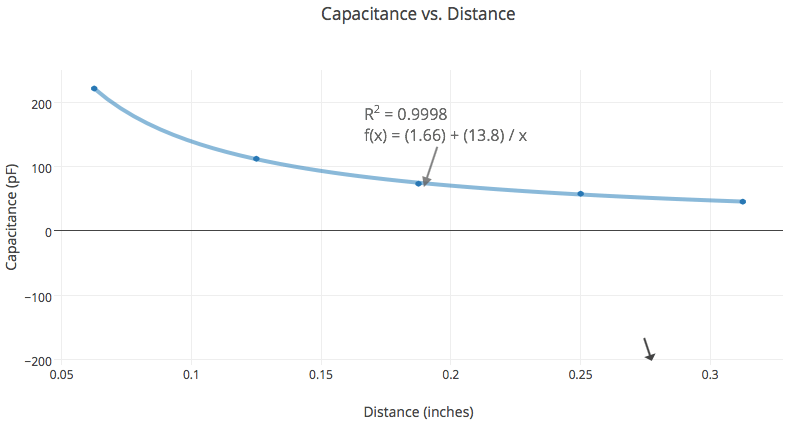After formally defining capacitance and deriving the various capacitance-related equations, I asked students a series of proportional reasoning questions: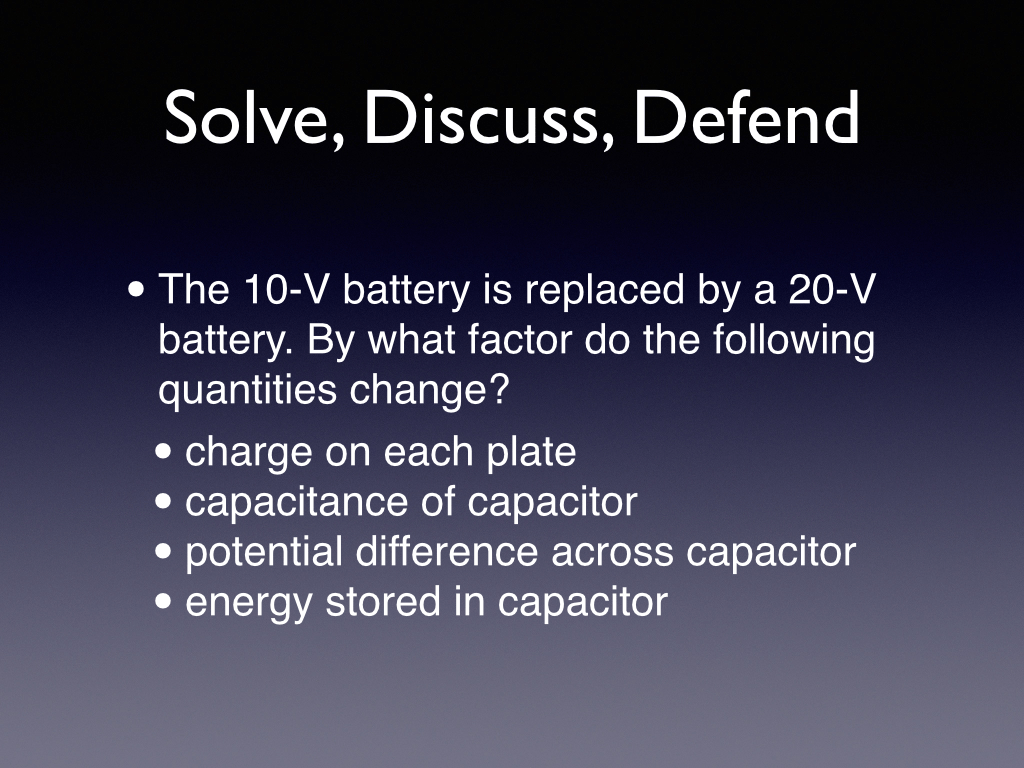The most thought-provoking follow-up question was after the last slide when I asked if the stored energy in the capacitor doubled, where did the energy come from?

## Day 55: Capacitor Paradigm Lab

Today, AP Physics 2 students completed the capacitor paradigm lab. The defined the purpose as to graphically and mathematically model the relationship between capacitance, area, and plate separation. They wanted to try different materials between the plates, but I only had acrylic available. I’ll try to get other materials for next year. Students used their best-fit-line equation from yesterday to measure the capacitance of the capacitors that they constructed from copper-clad circuit boards and acrylic.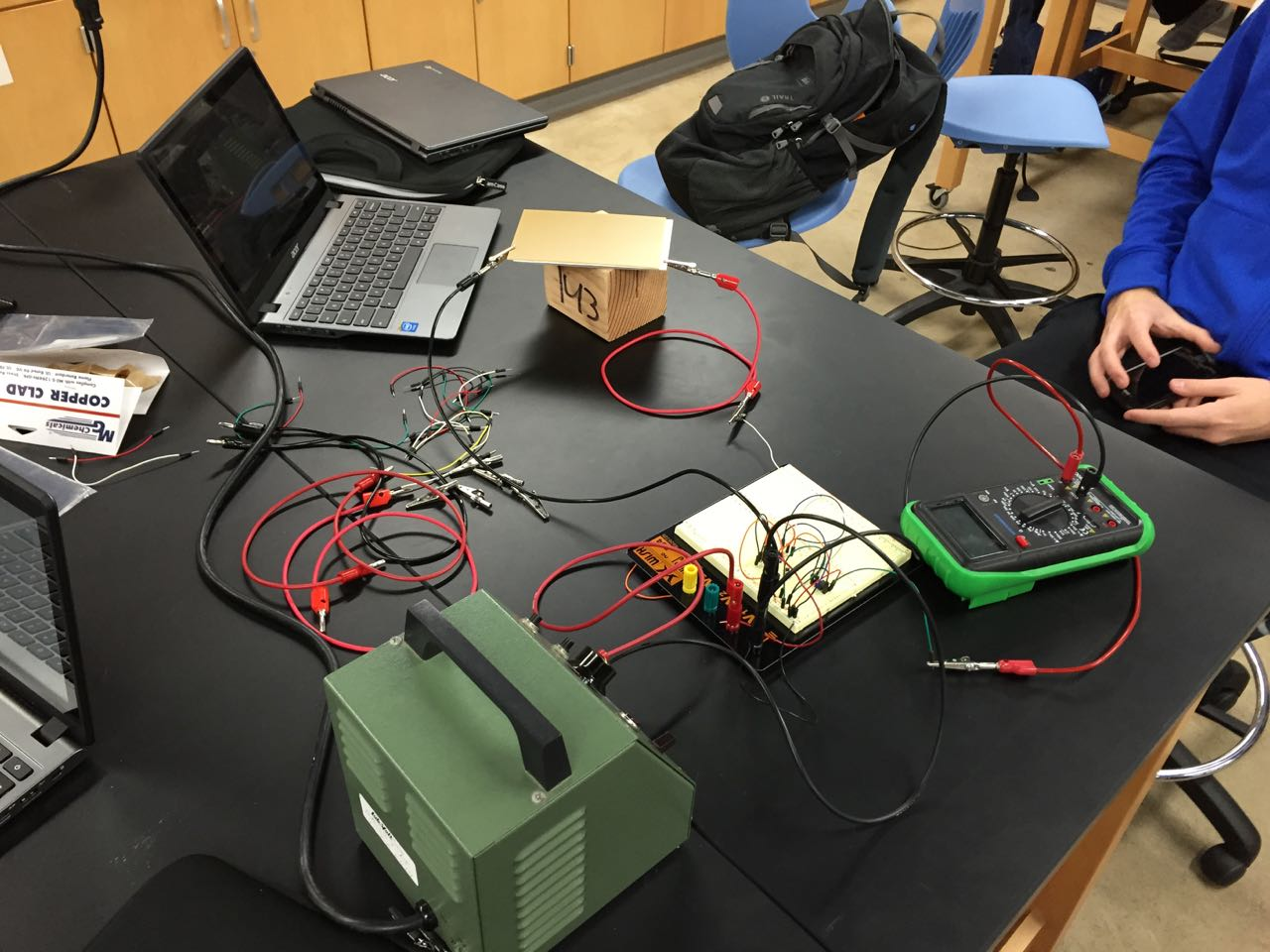## Day 54: Characterizing the Capacitance of the Circuit

Today, AP Physics 2 students quickly reconstructed the circuit from yesterday. Since the internal capacitance of this circuit is on the order of the capacitance of the parallel plate capacitors that they will build. Therefore, it is important to characterize this circuit to determine this internal capacitance. Characterizing the measurement apparatus is emerging as a theme in our labs. Students performed a similar characterization in the heat engine lab to determine the volume of the gas. In this case, students plotted capacitance vs. period and examined the intercept to determine the internal capacitance.## Day 53: Circuit to Measure Capacitance

Today, AP Physics 2 students start an extended lab to model the characteristics that determine the capacitance of a parallel plate capacitance. Before they can do that, they need a way to measure the capacitance of a capacitor. I shared with students a simple circuit I saw at the local AP Summer Institute from Patrick Polley that produces a signal whose period is proportional to the capacitance of the capacitor. For know student will treat this circuit as a black box; in the next unit, they will understand how it works. Students needed some time to brush up on their breadboard and circuits skills. So, they only had time to build the circuit not use it. We will build it again tomorrow!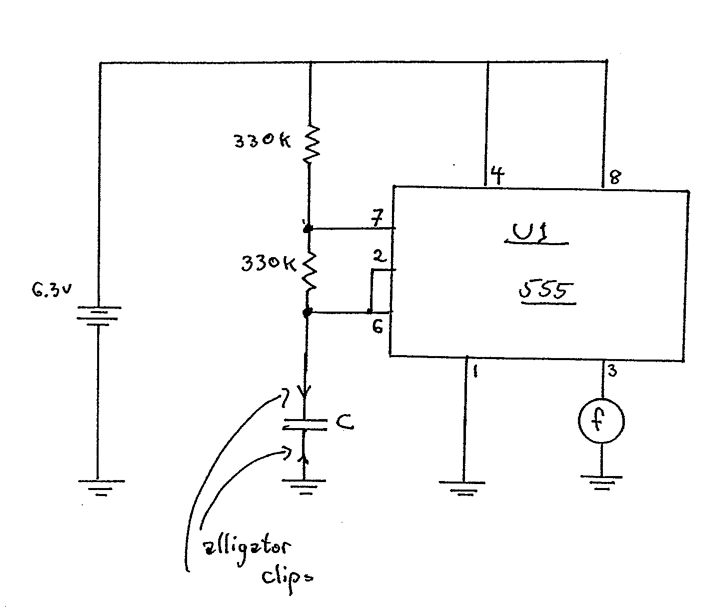## Day 52: Electric Potential Surfaces

Today, AP Physics 2 explored the resulting visual from yesterday’s mapping electric potential lab. We focused on visualizing the electric potential surface and continued to make an extended analogy between electric potential surfaces and equipotential lines and topographic maps and contour lines. Finally, we connected the magnitude of the electric field to the “steepness” (really gradient) of the electric potential surface.

I shared how an elevation plot of the electric potential surface can help visualize the electric potential and electric field. I shared xkcd’s Gravity Wells poster as an example of an elevation plot for gravitational potential.

The 3D electric potential plot of the conducting sheet with the closed conducting surface in the middle turned out fantastic. I nicely demonstrated that the electric potential within the closed conducting surface is constant and, therefore, the electric field is zero.##electrostatics ##representations

## Day 51: Mapping Electric Potential Lab

Today, AP Physics 2 students completed the Mapping Electric Potential Lab from Vernier’s Advanced Physics Beyond Mechanics lab manual. I purchased a conductive pen this year and modified on of the conductive sheets with parallel plates to include a closed conducting surface in the middle of the sheet. The 3D and 2D potential plots captured by the students look excellent. I’m especially interested in how the one with the closed conducting surface looks.##electrostatics